Here are my two circuits, their documentation (stills and video) and their calculations. Not sure if they’re all right, got a bit confused so looking forward to clarify this in class today. Had fun lighting things up though!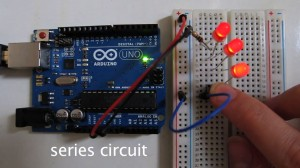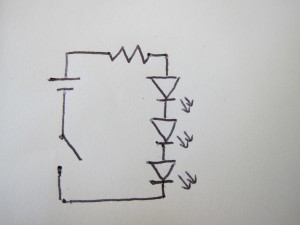V = 5/3 = 1.666…

R = 100

I = 1.666…/100

= 0.01666…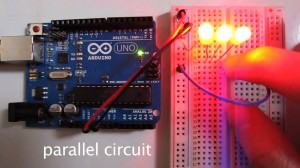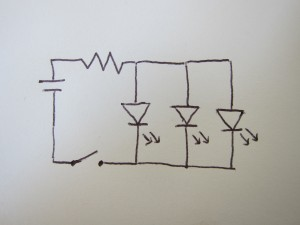V = 5

R = 100

I = 5/100

= 0.05

Video: Circuits

# Assignment 5: Circuits

Here is the diagram and calculations for my circuits. Was still confused about exactly how much resistance to use for what.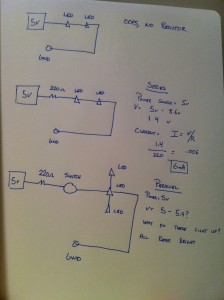And Vimeo links to both circuits: Circuit 1 , Circuit 2

I’ve been looking forward to using Arduino in projects for a long time, so it was nice to get started last class. I like the hands-on nature of physical computing and the fact that you can see the immediate result of your work, which is a change from Processing.

I’m still new to making circuits and using electricity, so it would be nice to understand those concepts a bit more. Why do we use certain resistors for certain components? How should this be calculated? Do you need to refer to the specs of all components?

# Circuits

parallel has three lights and series has two lights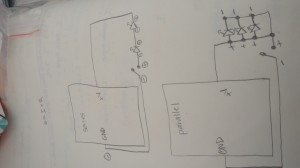I understand the equations involving voltage, current, and resistance:  V = I * R and I was powering my circuit with 6 volts and no resistors but I’m not sure how much resistance each LED takes and also how much the switch takes.  Also, I am not sure why I can’t get the series to light three LEDs.  It will only light 2.  Other than that, the pace and level of understanding for me is good.

Videos:

https://vimeo.com/51080557

https://vimeo.com/51080556

# Assignment6

Parallel and Series Circuit(Vimeo)

Schematic and Calculation (JPG)

Parallel and Series Circuit (Github)

Github Text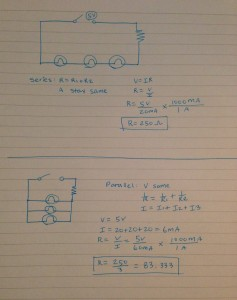I’m excited to make a something with LED but I’m still confused about it.
I didn’t know where to put the wires correctly that I burnt two of the bulbs.
Also, I don’t know if my calculations for voltage, resistance, and current is right.

# Parallel vs. Serial

Hi everybody,

Here are my two drawing for a parallel and Serial diagram.
I could not make my serial work with three(so here it is with two), also the reason why I calculated with two LED’s.

I went with the average volt of an LED as 2.2V, I was inspired by the tutorial(Jennifer:),

Also here is my git hub link to them in action: Parallel  And Serial

I’m very excited to start on making weird, blinking noisy stuff soon, I got to light sensors I really want to make something out of.

# LEDs

Hi, ya’ll,

Here are the links to my videos, plus my schematic and calculations.

I think this will be fun—I have zero experience with this, and I’m looking forward to learning all about it. I have some questions that came up while doing this assignment, and I’d love to talk about them in class, if others want to, too:

• I built a circuit—not posted—that used two LEDs, each of which had a forward voltage of 3.0v, in a series. Why did they light up at all? Why didn’t the 6.0v total between the LEDs eat up the entire voltage coming from the power supply (5v)? Likewise, I posted a picture of a three-LED series circuit using LEDs with a forward voltage of 1.8, and I’m not sure why that lit up.
• I’d like to talk more about the calculations in a series. Say you put four LEDs in a series with no resistor. There shouldn’t be enough voltage to light them. Are they effectively acting as resistors, or is it possible to not have enough voltage to light them, but still to have enough current to blow them out?
• What is the Vin pin in the Arduino?
• Where do watts come in to all this? What does it mean to have a 1/4 or 1/6 watt resistor?

Here is a video of three LEDs in parallel. Here are two in series. And here are three in series. The last ones are very dim, so the demonstration is in the dark.

Finally, here is the schematic with the calculations (voltage is 5v total and resistance is 200 ohms on all)—note: I updated this picture to show correct labeling of the circuits: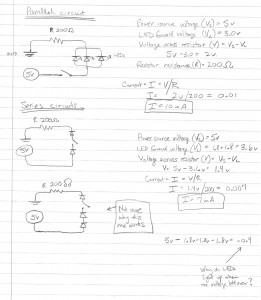The text of this post (minus the links) is also on github.

Looking forward to tomorrow’s class!

Jennifer

# circuits assignment

I put all my work into git hub, and here is the link.

# Assignment5

I uploaded 3 videos in vimeo

Parallel Circuit

Series Circuit

Parallel+Series circuit

Schematics + Calculations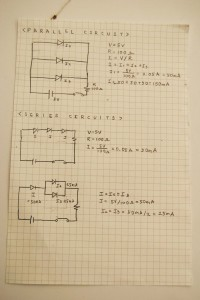If I calculate with LED resistor,

every LED has resistor and my LED resistor is 3V so

1. Current of first parallel circuit will be

5v-3v=2v

I=V/R

I=2v/100ohm

20mA+20mA+20mA=60mA

2. current of second series circuit will be 20mA.

3. current of third parallel and series circuit will be

I=20mA

I2=10mA

I3=10mA

I am taking Physical Computing so it was not really new and it is fun. From Pcom class everyone is good at arduino and the progress of class is fast

so I had hardtime to catch up. I think this cclab with arduino will really helpful to understand circuit.

Also I haven’t calculated ohm’s law before so it makes me to understand more about resistor voltage and current.